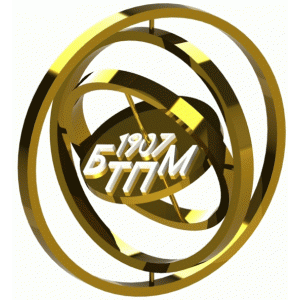# Department of STAM

Control problems for full-time students

 Problem on strength of materials (SМ-1) Problem on strength of materials (SМ-9) Problem on strength of materials (SМ-2) Problem on strength of materials (SМ-10) Problem on strength of materials (SМ-3) Problem on strength of materials (SМ-11) Problem on strength of materials (SМ-4) Problem on strength of materials (SМ-12) Problem on strength of materials (SМ-5) Problem on strength of materials (SМ-13) Problem on strength of materials (SМ-6) Problem on strength of materials (SМ-14) Problem on strength of materials (SМ-7) Problem on strength of materials (SМ-15) Problem on strength of materials (SМ-8)

 Problem on theoretical mechanics (ТМ-1) Problem on theoretical mechanics (ТМ-6) Problem on theoretical mechanics (ТМ-2) Problem on theoretical mechanics (ТМ-8) Problem on theoretical mechanics (ТМ-3) Problem on theoretical mechanics (ТМ-9) Problem on theoretical mechanics (ТМ-4) Problem on theoretical mechanics (ТМ-10) Problem on theoretical mechanics (ТМ-5)

Example of solution of the problem TM-9 part 1 .doc .docx .pdf part 2 .doc .docx .pdf
Control problems for Extramural Students
Control problems for Foreign students

сайт статистикиarrow_upward